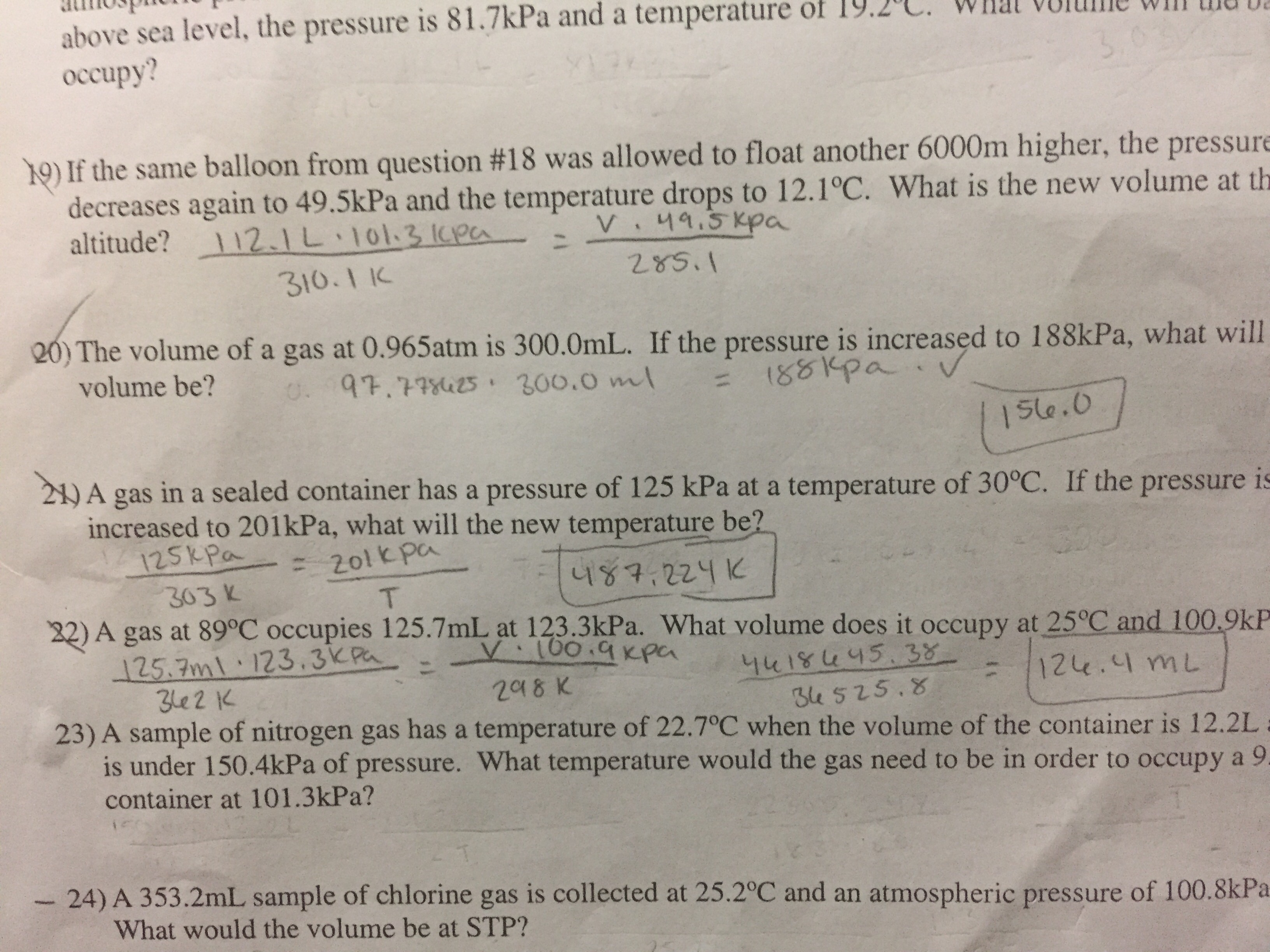# above sea level, the pressure is 8 1.7kPa and a temperature of 19.2℃ what volume will th occupy? )01f the same balloon from question #18 was allowed to float another 6000m higher, the pressure decreases again to 49.5kPa and the temperature drops to 12.1°C. What is the new volume at th altitude?一112.1 L-1013 ken -- v:ngsepa 310.1 IC The volume of a gas at 0.965atm is 300.0mL. If the pressure is increased to 1 88kPa, what will volume be? Ste.O 21) A gas in a sealed container has a pressure of 125 kPa at a temperature of 30°C. If the pressure is increased to 201kPa, what will the new temperature be? 25k Pa 201 kpa ule t 니ya.zzy K 32) A gas at 89c occupies 125.7mL, at 123.3kPa. What volume does it occupy at 25°C and 100.9kP 303 V 25.7ml 123.3KPa Juisu 15.38 e 525.8 e2 20 8 K 12e.ImL 23) A sample of nitrogen gas has a temperature of 22.7°C when the volume of the container is 12.2L is under 150.4kPa of pressure. What temperature would the gas need to be in order to occupy a 9 container at 101.3kPa? - 24) A 353.2mL sample of chlorine gas is collected at 25.2°C and an atmospheric pressure of 100.8kPa What would the volume be at STP?

Questionhelp_outlineImage Transcriptioncloseabove sea level, the pressure is 8 1.7kPa and a temperature of 19.2℃ what volume will th occupy? )01f the same balloon from question #18 was allowed to float another 6000m higher, the pressure decreases again to 49.5kPa and the temperature drops to 12.1°C. What is the new volume at th altitude?一112.1 L-1013 ken -- v:ngsepa 310.1 IC The volume of a gas at 0.965atm is 300.0mL. If the pressure is increased to 1 88kPa, what will volume be? Ste.O 21) A gas in a sealed container has a pressure of 125 kPa at a temperature of 30°C. If the pressure is increased to 201kPa, what will the new temperature be? 25k Pa 201 kpa ule t 니ya.zzy K 32) A gas at 89c occupies 125.7mL, at 123.3kPa. What volume does it occupy at 25°C and 100.9kP 303 V 25.7ml 123.3KPa Juisu 15.38 e 525.8 e2 20 8 K 12e.ImL 23) A sample of nitrogen gas has a temperature of 22.7°C when the volume of the container is 12.2L is under 150.4kPa of pressure. What temperature would the gas need to be in order to occupy a 9 container at 101.3kPa? - 24) A 353.2mL sample of chlorine gas is collected at 25.2°C and an atmospheric pressure of 100.8kPa What would the volume be at STP? fullscreen

### Want to see the step-by-step answer?